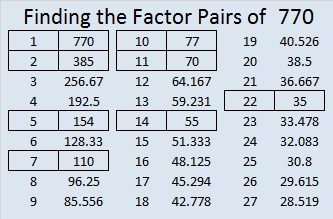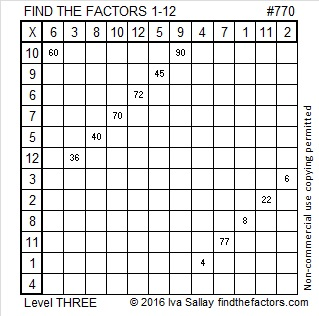# 770 and Level 3

• 770 is a composite number.
• Prime factorization: 770 = 2 x 5 x 7 x 11
• The exponents in the prime factorization are 1, 1, 1, and 1. Adding one to each and multiplying we get (1 + 1)(1 + 1)(1 + 1)(1 + 1) = 2 x 2 x 2 x 2 = 16. Therefore 770 has exactly 16 factors.
• Factors of 770: 1, 2, 5, 7, 10, 11, 14, 22, 35, 55, 70, 77, 110, 154, 385, 770
• Factor pairs: 770 = 1 x 770, 2 x 385, 5 x 154, 7 x 110, 10 x 77, 11 x 70, 14 x 55, or 22 x 35
• 770 has no square factors that allow its square root to be simplified. √770 ≈ 27.74887.Here is a puzzle for you to solve:Print the puzzles or type the solution on this excel file: 12 Factors 2016-02-25

————————————-

Because 5 is one of its factors, 770 is the hypotenuse of a Pythagorean triple, and 462² + 616² = 770².

770 is the sum of the squares of three consecutive numbers: 15² + 16² + 17² = 770.

770 can also be written as the sum of three squares seven other ways:

• 27² + 5² + 4² = 770
• 25² + 12² + 1² = 770
• 25² + 9² + 8² = 770
• 24² + 13² + 5² = 770
• 23² + 15² + 4² = 770
• 20² + 19² + 3² = 770
• 20² + 17² + 9² = 770

770 is palindrome MM in Base 34 (M = 22 base 10); note that 22(34) + 22(1) = 770.

————————————-## 4 thoughts on “770 and Level 3”

1.Opinionated Man

I’ll be back soon with my kid’s homework! 😉

•ivasallay

I’m curious about the homework! What is it?

•Opinionated Man

Basic stuff for now since they are young, but it will get harder!

2.ivasallay

And more fun, too.

This site uses Akismet to reduce spam. Learn how your comment data is processed.